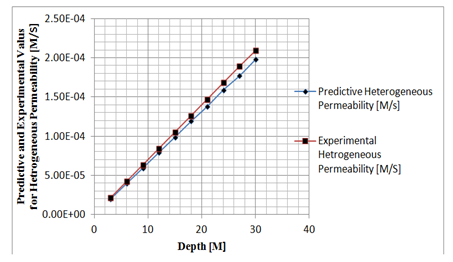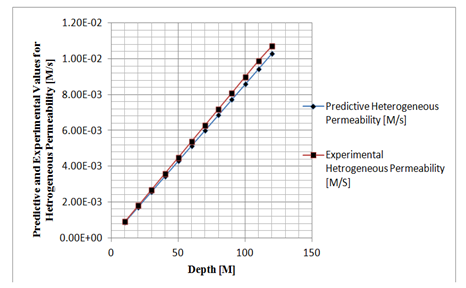##### MOJ
###### eISSN: 2572-8520# Civil EngineeringResearch Article Volume 4 Issue 4

# Predicting heterogeneous permeability coefficient pressured by heterogeneous seepage on coarse deposition

#### Eluozo SN,1 function clickButton(){ var name=document.getElementById('name').value; var descr=document.getElementById('descr').value; var unCopyslNo=document.getElementById('unCopyslNo').value; document.getElementById("mydiv").style.display = "none"; $.ajax({ type:"post", url:"https://medcraveonline.com/captchaCode/server_action", data: { 'name' :name, 'descr' :descr, 'unCopyslNo': unCopyslNo }, cache:false, success: function (html) { //alert('Data Send');$('#msg').html(html); } }); return false; } Verify Captcha Regret for the inconvenience: we are taking measures to prevent fraudulent form submissions by extractors and page crawlers. Please type the correct Captcha word to see email ID.function refreshPage(){ $("#mydiv").load(location.href + " #mydiv"); }$(document).ready(function () { //Disable cut copy paste $('#msg').bind('cut copy paste', function (e) { e.preventDefault(); }); //Disable mouse right click$("#msg").on("contextmenu",function(e){ return false; }); }); .noselect { -webkit-touch-callout: none; /* iOS Safari */ -webkit-user-select: none; /* Safari */ -khtml-user-select: none; /* Konqueror HTML */ -moz-user-select: none; /* Firefox */ -ms-user-select: none; /* Internet Explorer/Edge */ user-select: none; /* Non-prefixed version, currently supported by Chrome and Opera */ cursor: none; } Oba AL2

1Department of Civil and Environmental Engineering, Gregory University Uturu (GUU), Abia State of Nigeria, Nigeria
2Department of Civil Engineering, Ken-Saro Wiwa Polytechnic Bori, Nigeria

Correspondence: luozo SN, Department of Civil and Environmental Engineering, Gregory University Uturu (GUU), Abia State of Nigeria

Received: May 11, 2018 | Published: August 23, 2018

Citation: Predicting heterogeneous permeability coefficient pressured by heterogeneous seepage on coarse deposition. MOJ Civil Eng. 2018;4(4):257-261. DOI: 10.15406/mojce.2018.04.00128

# Abstract

This paper monitor coefficient of permeability pressured by heterogeneous seepage in coarse depositions, previous studies carried out express permeability coefficient influences by Void Ratio on Heterogeneous Compressibility of Fine Sand Formation in Obio-Akpor, that study discuses void ratios as a pressure to the rate of compressibility of litho structures of that stratum in that study location, the lithology determine coefficient of the stratum permeation in fluid flow in such deltaic depositions. But for these study it focuses on heterogeneous seepage force under heterogeneous permeability coefficient on depositions of coarse sand, other study variables from other slight depositions were observed to influences the permeability coefficient, several experts has applied experimental procedures to determine permeability coefficient and its rates of seepage force in some strata, there is no literature that has monitor the permeation of soil strata with analytical solutions from seepage force influences, the study evaluates the refection of heterogeneity structured strata of coarse deposition, it also monitor other slight deposited formation reflected on the variation of permeability in the deltaic environment. From the simulation, increase of permeability where observed, these express the litho structure of coarse deposition in coastal regions, while some simulation values explain the reality of deltaic deposition that are not in coastal environments. Finally, other simulation reflects some structured strata that developed lower permeations, these areas will definitely developed low yield of phreatic depositions or accumulation of contaminant in pollution transport. The study were subjected to simulations, these values predict the heterogeneous deposition of permeability under seepage influences in deltaic formations, and the predicted values were compared with experimental data for model validation, and both parameters express favorable fits.

Keywords: permeability coefficient, heterogeneous, Seepage and coarse formation

# Introduction

Among hydro-geological, geological, and geotechnical problems in earth dams, seepage is a major concern associated with dams. Because it threatens dam stability and may cause unforeseen failure.1-6 Many methods such as using of cut off wall and injection to avoid seepage are proposed. Otherwise the prediction of seepage discharge through earth dam can be useful for seepage controls. Fealeh Khaseh dam located in Zanjan province, west of Iran, is used as a case study in this paper. Although there are many established theoretical relation between seepage and soil properties their association and evaluation of seepage requires system identification techniques.7-8 The interdependency of factors involved in such problems prevents the use of regression analysis and demands a more extensive and sophisticated method. The Group Method of Data Handling (GMDH) type of artificial neural networks (ANN) optimized by Genetic Algorithms (GAs) can be used for complex systems modeling, where unknown relationships exist between the variables, without having a specific knowledge of the processes. In recent years, the use of such self-organizing neural networks has led to successful application of the GMDH-type of algorithm in geotechnical sciences.9-12 This situation continues gradually until difference between water potential in up and down stream cause moving particles from porosity and starting piping suddenly. This event is named as seepage. Lakshmi13 in theory, seepage is named gravitation water which flow into soil particles porosity in result of gravity. Seepage in dam is similar to flow in an open channel because their free surface and atmosphere pressure.14-16 But, underground water is including two sections liquid/solid; and, flow velocity and discharge are controlled by soil permeability. A method to display flow in body and foundation of earth dam is Flow net. The primary line in flow net is named as phreatic line which restricts seepage.17,18

# Governing equation

$\frac{Av+As}{Av}\frac{\partial ks}{\partial L}-\frac{\partial {k}^{2}s}{\partial t}+\frac{\left(Av+As\right)}{AvL}+\frac{\partial ks}{\partial L}V\frac{\left(Vv+Vs\right)}{Vv}$  (1)

$\frac{\partial ks}{\partial L}={S}^{1}ks\left(L\right)-Sks\left(o\right)$ (2)

$\frac{\partial ks}{\partial t}={S}^{1}ks\left(L\right)-Sks\left(o\right)$(3)

$\frac{\partial \Phi s}{\partial L}={S}^{1}ks\left(L\right)-Sks\left(o\right)$ (4)

${S}^{1}Vks\left(L\right)-\frac{Av+As}{Av}\left[{S}^{1}\left(L\right)-{S}^{1}ks\left(o\right)\right]+V\frac{\left(Av+As\right)}{AvL}\left[{S}^{1}ks\left(t\right)-{S}^{1}ks\left(z\right)\right]$ (5)

${S}^{1}\left(L\right)-ks\left(o\right)=\frac{Av+As}{Av}{S}^{1}ks\left(o\right)-\frac{\left(Av+As\right)}{AvL}ks\left(o\right)$   (6)

$V\frac{\left(Av+As\right)}{AvL}Vs\left(L\right)-V\frac{\left(Av+As\right)}{AvL}SVs\left(o\right)$ (7)

$\Phi \frac{\left(Vv+Vs\right)}{Vv}ks\left(z\right)-Sks\left(o\right)$  (8)

Let $ks\left(o\right)=0$

We have

${S}^{1}\left(L\right)-\frac{\left(Av+As\right)}{Av}ks\left(o\right)+k\frac{\left(Av+As\right)}{AvL}ks\left(t\right)+k\frac{\left(Vv+Vs\right)}{Vv}{S}^{1}ks\left(L\right)$ (9)

$\Phi {S}^{1}\left(L\right)=\frac{1}{S}\left[\frac{\left(Av+As\right)}{Av}\Phi s\left(L\right)-\Phi \frac{\left(Av+As\right)}{AvL}{S}^{1}+\Phi \frac{\left(Vv+Vs\right)}{Vv}{S}^{1}\Phi s\left(L\right)\right]$ (10)

$kS\left(L\right)=\frac{1}{{S}^{1}}\left[\frac{\left(Av+As\right)}{Av}ks\left(L\right)-k\frac{\left(Av+As\right)}{AvL}{S}^{1}+k\frac{\left(Vv+Vs\right)}{Vv}\right]$ (11)

$k{S}^{1}\left(L\right)=\frac{\frac{\left(Av+As\right)}{Av}ks\left(L\right)-k\frac{\left(Av+As\right)}{AvL}ks\left(t\right)+k\frac{\left(Vv+Vs\right)}{Vv}}{{S}^{1}}$  (12)

$kS\left(L\right)=\frac{\left(Av+As\right)}{Av}{S}^{1}ks\left(L\right)+k\frac{\left(Vv+Vs\right)}{Vv}kS\left(t\right)$(13)

$kS\left(L\right)=\frac{\frac{\left(Av+As\right)}{Av}ks\left(L\right)=k\frac{\left(Av+As\right)}{AvL}ks\left(L\right)+k\frac{\left(Vv+Vs\right)}{Vv}}{S}$(14)

$kS\left(L\right)=\left[\frac{\left(Av+As\right)}{Av}+k\frac{\left(Av+As\right)}{AvL}+k\frac{\left(Vv+Vs\right){S}^{1}}{Vs}\right]\text{\hspace{0.17em}}kS\left(t\right)$ (15)

${S}^{1}kS\left(L\right)=\left(\frac{\left(Av+As\right)}{Av}+k\frac{\left(Av+As\right)}{AvL}+k\frac{\left(Vv+Vs\right){S}^{1}}{Vs}\right)kS\left(t\right)$(16)

$\frac{Av+As}{Av}+k\frac{\left(Av+As\right)}{AvL}+k\frac{\left(Vv+Vs\right){S}^{1}}{Vs}$   (17)

$kS\left(L\right)\frac{{S}^{1}\left(L\right)}{\frac{Av+As}{Av}+k\frac{\left(Av+As\right)}{AvL}+k\frac{\left(Vv+Vs\right){S}^{1}}{Vs}}$  (18)

Furthermore, considering the boundary condition, we have the following

At $t=0{V}^{1}S\left(o\right)=VS\left(o\right)=0$

$\frac{Av+As}{Av}kS\left(L\right)-k\frac{\left(Av+As\right){S}^{1}}{AvL}kS\left(L\right)+k\frac{\left(Vv+Vs\right)}{Vv}kS\left(t\right)=0$  (19)

$kS\left(L\right)=\frac{Av+As}{Av}-k\frac{\left(Av+As\right)}{AvL}+k\frac{\left(Vv+Vs\right)}{Vs}$  (20)

Considering the following boundary conditions when

At $t>0{\Phi }^{1}S\left(o\right)=\Phi S\left(o\right)$

Applying the boundary condition into this equation

$\frac{Av+As}{Av}kS\left(L\right)-\frac{\left(Av+As\right)}{Av}+k\frac{\left(Av+As\right)}{AvL}kS\left(L\right)+k\frac{\left(Av+As\right)}{AvL}kSo-S\left(L\right)+V\frac{\left(Vv+Vs\right)}{Vv}VS\left(t\right)+V\frac{\left(Vv+Vs\right)}{Vv}VSo+S\left(t\right)$ (21)

$\frac{\left(Av+As\right)}{Av}\left(L\right)-\frac{\left(Av+As\right)}{AvL}kS\left(o\right)\frac{\left(Av+As\right)}{Av}kSo+k\frac{\left(Vv+Vs\right)}{Vv}kSo$  (22)

$kS\left(L\right)=\left[\frac{Av+Ass}{Av}=k\frac{\left(Av+As\right)}{Av}+k\frac{\left(Av+As\right)}{AvL}+k\frac{\left(Vv+Vs\right)}{Vv}\right]kSo$(23)

$kS\left(L\right)=\frac{Av+Ass}{Av}-\frac{\left(Av+As\right)}{Av}-k\frac{\left(Av+As\right)}{AvL}+k\frac{\left(Vv+Vs\right)}{Vv}kSo$ (24)

$kS\left(L\right)=\frac{\frac{\left(Av+Ass\right)}{Av}-\frac{\left(Av+As\right)}{Av}-k\frac{\left(Av+As\right)}{AvL}+k\frac{\left(Vv+Vs\right)}{Vv}VSo}{\frac{\left(Av+As\right)}{Av}S-\frac{\left(Av+As\right)}{Av}+k\frac{\left(Vv+Vs\right)}{Vv}}$ (25)

Applying quadratic equation to determine denominator for the equation

$\frac{Av+As}{Av}-k\frac{\left(Av+As\right)}{AvL}+{k}^{2}\frac{\left(Vv+Vs\right)}{Vv}=0$ (26)

$s=\frac{-b±\sqrt{{b}^{2}-4ac}}{2ac}$(27)

Where$a=\frac{Av+As}{Av}$, $b={k}^{2}\frac{\left(Av+As\right)}{AvL}$ and $c=k\frac{\left(Vv+Vs\right)}{Vv}kS$

For simplicity denoting the expressed functions parameter of the following

Let $\frac{Av+As}{Av}=Q,$$V\frac{\left(Av+As\right)}{AvL}={\lambda }^{2}$ and $k\frac{\left(Vv+Vs\right)}{Vv}=\alpha$

Integrating the express parameters into the quadratic function we have:

$S=\frac{-{\lambda }^{2}±\sqrt{{\lambda }^{2}-4k\alpha VS}}{2kVS}$ (28)

$\left[{S}_{1}=\frac{{\lambda }^{2}+\sqrt{{\lambda }^{2}-4k\alpha VS}}{2kVS}\right]\left[{S}_{2}=\frac{{\lambda }^{2}-\sqrt{{\lambda }^{2}-4k\alpha VS}}{2kVS}\right]$
$\mathcal{l}{\text{\hspace{0.17em}}}^{\text{\hspace{0.17em}}\left[\frac{\sqrt{{\lambda }^{2}\text{\hspace{0.17em}}-\text{\hspace{0.17em}}4Q\alpha VS}}{2kVS}\right]\text{\hspace{0.17em}}t\text{\hspace{0.17em}}\text{\hspace{0.17em}}\left[\frac{\text{\hspace{0.17em}}\lambda \text{\hspace{0.17em}}\sqrt{{\lambda }^{2}\text{\hspace{0.17em}}-\text{\hspace{0.17em}}4Q\alpha VS}}{2kVS}\right]t}$ (29)

The inverse Laplace of the equation yield

$VS\left(L\right)=\left[\frac{Q}{t}+Q+\lambda +\alpha \right]VSo{\mathcal{l}}^{\left[\frac{\lambda +\sqrt{{\lambda }^{2}+4Q\alpha VS}}{kVS}\right]t}$

$\left[\left[\frac{\lambda -\sqrt{{\lambda }^{2}-4k\alpha VS}}{2kVS}\right]\right]t-\left[\frac{\lambda -\sqrt{{\lambda }^{2}+4k\alpha VS}}{2kVS}\right]t$ (30)

$VS\left(L\right)=\left[\frac{\lambda }{{t}^{2}}VSo\right]\left[\left[\frac{\lambda +\sqrt{{\lambda }^{2}-4k\alpha VS}}{2kVS}\right]\right]{\mathcal{l}}^{\left[\frac{\lambda \sqrt{{\lambda }^{2}-4Q\alpha VS}}{2kVS}\right]t}$

$\mathcal{l}{\text{\hspace{0.17em}}}^{\text{\hspace{0.17em}}\left[\frac{\lambda \text{\hspace{0.17em}}\sqrt{{\lambda }^{2}\text{\hspace{0.17em}}-\text{\hspace{0.17em}}4k\alpha VS}}{2kVS}\right]\text{\hspace{0.17em}}t\text{\hspace{0.17em}}\text{\hspace{0.17em}}-\left[\frac{\text{\hspace{0.17em}}\lambda \sqrt{{\lambda }^{2}\text{\hspace{0.17em}}-\text{\hspace{0.17em}}4k\alpha VS}}{2kVS}\right]t}$  (31)

At this point $VSo=0t\ne 0$

For equation (30) at $t=0VS\left(o\right)=VSo$ we have

$VSo=\left[\left(k+\lambda +\alpha \right)VSo\left(1+1+1\right)\right]=0=\left(k+\lambda +\alpha \right)$

Hence $Q+\lambda +\alpha =0$

Equation (31) becomes

$VS\left(L\right)-VSo\left[\frac{\lambda }{{t}^{2}}+2\right]\left[\frac{\lambda +\sqrt{{\lambda }^{2}-4k\alpha VS}}{2kVS}\right]t\left[\frac{\lambda \sqrt{{\lambda }^{2}-4k\alpha VS}}{2kVS}\right]$ (32)

We recall that $Hence\text{\hspace{0.17em}}\text{\hspace{0.17em}}{\mathcal{l}}^{x}\text{\hspace{0.17em}}+\text{\hspace{0.17em}}{\mathcal{l}}^{-x\text{\hspace{0.17em}}}\text{\hspace{0.17em}}=\text{\hspace{0.17em}}\text{\hspace{0.17em}}2\mathrm{Cos}\text{\hspace{0.17em}}x,$so that equation (32) can be expressed as:

$kS\left(L\right)-\left[\lambda +2\right]kSoCos\left[\frac{\lambda \sqrt{{\lambda }^{2}-4k\alpha VS}}{2kVS}\right]t$ (33)

# Materials and methods

Standard laboratory experiment where performed to monitor the rate of permeability depositions at different formation, the soil depositions of the strata were collected in sequences base on the structural deposition at different study area, this samples collected at different location generated variation of permeability coefficient at different depth producing different coefficient of permeability through its pressure flow at the lower end using falling head permeability test at different strata, the experimental result are applied to be compared with theoretical values for model validation.

# Results and discussion

Results and discussion are presented in tables including graphical representation of heterogeneous permeability coefficient at different Depth and Time (Tables 1–6).

 Time per day Predictive heterogeneous permeability [M/s] Experimental heterogeneous permeability [M/S] 10 1.95E-02 2.00E-02 20 3.90E-02 4.00E-02 30 5.90E-02 6.00E-02 40 7.80E-02 8.00E-02 50 9.75E-02 1.00E-01 60 1.17E-01 1.20E-01 70 1.37E-01 1.40E-01 80 1.56E-01 1.60E-01 90 1.76E-01 1.80E-01 100 1.95E-01 2.00E-01

Table 1 Predictive and experimental values of heterogeneous permeability at different time

 Depth [M] Predictive heterogeneous permeability [M/s] Experimental heterogeneous permeability [M/S] 3 1.32E-05 1.20E-05 6 2.65E-05 2.40E-05 9 3.98E-05 3.60E-05 12 5.32E-05 4.80E-05 15 6.64E-05 6.00E-05 18 7.98E-05 7.20E-05 21 9.30E-05 8.40E-05 24 1.06E-04 9.60E-05 27 1.19E-04 1.08E-04 30 1.33E-04 1.20E-04

Table 2 Predictive and experimental values of heterogeneous permeability at different depth

 Depth [M] Predictive heterogeneous permeability [M/s] Experimental heterogeneous permeability [M/S] 3 1.32E-06 1.40E-06 6 2.10E-06 2.60E-06 9 3.98E-06 3.80E-06 12 5.32E-06 5.00E-06 15 6.64E-06 6.20E-06 18 7.78E-06 7.40E-06 21 9.30E-06 8.60E-06 24 1.06E-05 9.80E-06 27 1.19E-05 1.10E-05 30 1.33E-05 1.22E-05

Table 3 Predictive and experimental values of heterogeneous permeability at different depth

 Depth [M] Predictive heterogeneous permeability [M/s] Experimental heterogeneous permeability [M/S] 3 1.97E-05 2.09E-05 6 3.95E-05 4.19E-05 9 5.92E-05 6.29E-05 12 7.91E-05 8.39E-05 15 9.88E-05 1.05E-04 18 1.19E-04 1.26E-04 21 1.38E-04 1.47E-04 24 1.59E-04 1.68E-04 27 1.77E-04 1.89E-04 30 1.98E-04 2.09E-04

Table 4 Predictive and experimental values of heterogeneous permeability at different depth

 Depth [M] Predictive heterogeneous permeability [M/s] Experimental heterogeneous permeability [M/S] 3 2.65E-05 2.80E-05 6 5.32E-05 5.50E-05 9 7.98E-05 8.20E-05 12 1.14E-04 1.09E-04 15 1.33E-04 1.36E-04 18 1.59E-04 1.63E-04 21 1.86E-04 1.90E-04 24 2.13E-04 2.17E-04 27 2.39E-04 2.44E-04 30 2.66E-04 2.71E-04

Table 5 Predictive and experimental values of heterogeneous permeability at different depth

 Time per day Predictive heterogeneous permeability [M/s] Experimental heterogeneous permeability [M/S] 10 8.58E-04 8.96E-04 20 1.71E-03 1.79E-03 30 2.57E-03 2.69E-03 40 3.43E-03 3.59E-03 50 4.29E-03 4.49E-03 60 5.15E-03 5.39E-03 70 6.00E-03 6.29E-03 80 6.86E-03 7.19E-03 90 7.72E-03 8.09E-03 100 8.58E-03 8.99E-03 110 9.44E-03 9.89E-03 120 1.03E-02 1.07E-02

Table 6 predictive and experimental values of heterogeneous permeability at different time

Figures 1–6 presented express the rate heterogeneous deposition of permeability in coarse formation in every location at the study area. All the trend from the figures express exponential phase trend, these are all in linear conditions were the lowest were observed at ten days at the depth of three metres, while the optimum are recorded at thirty six metres depth at the period of hundred and twenty days, but the simulation values are heterogeneous from the permeability coefficient values. More so, based on the concept of simulation, the predictive values were observed to have reflected from heterogeneous deposition of the strata as experienced from the figures above. The values in some figure are reflecting low permeation varying between 1.32E-06 -1.00E-02.These implies that the structure of the strata establish predominant variation of coarse heterogeneity in the study environment, while in some area develop predominance with low depositions low coefficient of permeability, these are base on the rate of predominant structure strata heterogeneity thus developed fluid velocity that cannot produce productive yield rate in phreatic zones. The predictive values from various figures were compared with experimental values for model validation, both parameters developed favorable fits.Figure 1 Predictive and experimental values of heterogeneous permeability at different time.Figure 2 Predictive and experimental values of heterogeneous permeability at different depth.Figure 3 Predictive and experimental values of heterogeneous permeability at different depth.Figure 4 Predictive and experimental values of heterogeneous permeability at different depth.Figure 5 Predictive and experimental values of heterogeneous permeability at different depth.Figure 6 Predictive and experimental values of heterogeneous permeability at different depth.

# Conclusion

The study has developed coefficient of permeability applying deterministic modeling application, several research determined coefficient of permeability using experimental procedure, there is no literature expect other modeling concept done by Eluozo,19 these was to monitor permeability coefficient under predominant effect from void ratio and compressibility of fine sand, the seepage force were observed from fracture between the porous rock deposition during disintegration or weathering period, this generated the heterogeneity of fluid flow in permeable coarse depositions, the developed model were subjected to simulation, these generated predictive values were compared with experimental values for model validation, the results from predictive values experienced linear flow with variation of permeability coefficient from the graphical representations, most values deposited lower permeability coefficient compared to others, the study generated permeability coefficient that can be experienced in deltaic deposition at different environments . Finally, other simulation reflects some structured strata that developed lower permeations, these areas will definitely developed low yield in phreatic depositions or accumulation of contaminant in pollution transport. The study was subjected to simulations; these values predict the heterogeneous depositions of permeability under seepage influences in deltaic formations.20-25

None.

# Conflicts of interest

The author declares there is no conflict of interest.